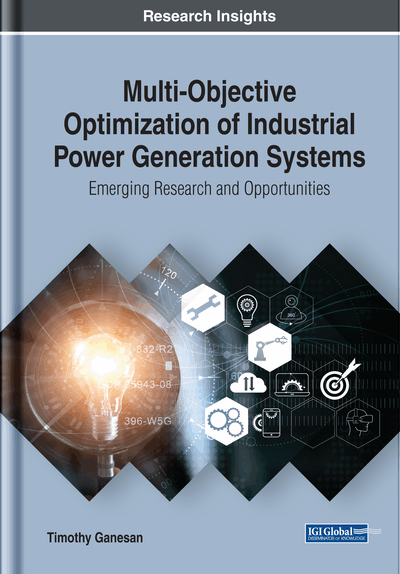# Biofuel Supply Chain Optimization Using Random Matrix Generators

DOI: 10.4018/978-1-7998-1710-9.ch006
OnDemand:
(Individual Chapters)
Available
\$29.50
No Current Special Offers

## Abstract

Supply chain problems are large-scale problems with complex interlinked variables. This sort of characteristic closely resembles structures often encountered in the nuclei of heavy atoms (e.g., platinum, gold or rhodium). Such structures are said to have the property of universality.
Chapter Preview
Top

## Random Matrix Theory

Random Matrix Theory (RMT) is a robust mathematical technique used to describe the behavior of complex systems. Due to its applicability to a vast range of systems, RMT is known to exhibit universality – a property of global symmetries shared by many systems within a certain symmetry class. In some sense RMT is a generalization of conventional statistical systems that often consists of matrices with fixed eigenvalues. In RMT, the eigenvalues themselves are statistically variant fitting into a probability distribution. This way RMT deals with the statistical behavior of the eigenvalues of random matrices. On the other hand, the random matrices have stochastic entries which are described by a distinct probability distribution function. Therefore in RMT there exists two probability distributions describing: the random matrix entries and the eigenvalue spread. The nearest neighbor spacing probability distribution (Schweiner et al., 2017) of eigenvalues is given by Wigner’s Surmise:

(6.1) where s is the eigenvalue spacing, Ai and Bi are constant parameters. The normalized spacing, s and the mean spacingis as follows:such that(6.2) whereis the nth eigenvalue sequentially such that. The first type of random matrices are those that are modeled based on complex quantum systems (which have chaotic classical counterparts). The index i represent systems falling into this type of RMT theory; whereby i = 1, 2 and 4 denotes the Gaussian Orthogonal Ensemble (GOE) (Krishnan et al., 2017), Gaussian Unitary Ensemble (GUE) (Arguin et al., 2017) and Gaussian Symplectic Ensemble (GSE) (Rehemanjiang et al., 2016) spacing distributions respectively (Schierenberg et al., 2012). These spacing distributions are given as follows:

## Complete Chapter List

Search this Book:
Reset